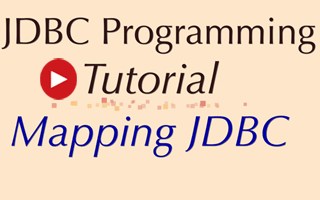# Mapping MySQL Data Types in Java

Data types of MySQL and Java programming language are not same, its need some mechanism for transferring data between an database using MySQL data types and a application using Java data types.# Mapping MySQL Data Types in Java

Data types of MySQL and Java programming language are not same, its need some mechanism for transferring data between an database using MySQL data types and a application using Java data types. We need to provide Java mappings for the common MySQL data types. We have to confirm that we have proper type information then only we can correctly store and retrieve parameters and recover results from MySQL statements.

There is no particular reason that the Java data type needs to be exactly isomorphic to the MySQL data type. For example, Java String don't precisely match any of the MySQL data CHAR type, but it gives enough type information to represent CHAR, VARCHAR or LONGVARCHAR successfully.

The following table represent the default Java mapping for various common MySQL data types:

 MySQL Type Java Type CHAR String VARCHAR String LONGVARCHAR String NUMERIC java.math.BigDecimal DECIMAL java.math.BigDecimal BIT boolean TINYINT byte SMALLINT short INTEGER int BIGINT long REAL float FLOAT double DOUBLE double BINARY byte [] VARBINARY byte [] LONGVARBINARY byte [] DATE java.sql.Date TIME java.sql.Time TIMESTAMP java.sql.Tiimestamp

Here is the video description:

1. CHAR, VARCHAR and LONGVARCHAR
MySQL data types CHAR, VARCHAR, LONGVARCHAR are closely related. CHAR represents a small, fixed-length character string, VARCHAR represents a small, variable-length character string, and LONGVARCHAR represents a large, variable-length character string. There is no need for Java programmer to distinguish these three MySQL data types. These can be expressed identically in Java. These data types could be mapped in Java to either String or char[]. But String seemed more appropriate type for normal use. Java String class provide a method to convert a String into char[] and a constructor for converting a char[] into a String.

The method ResultSet.getString allocates and returns a new String. It is suitable for retrieving data from CHAR, VARCHAR and LONGVARCHAR fields. This is suitable for retrieving normal data, but LONGVARCHAR MySQL type can be used to store multi-megabyte strings. So that Java programmers needs a way to retrieve the LONGVARCHAR value in chunks. To handle this situation, ResultSet interface have two methods for allowing programmers to retrieve a LONGVARCHAR value as a Java input stream from which they can subsequently read data in whatever size chunks they prefer. These methods are getAsciiStream and getCharacterStream, which deliver the data stored in a LONGVARCHAR column as a stream of ASCII or Unicode characters.2. NUMERIC and DECIMAL
The NUMERIC and DECIMAL MySQL data types are very similar. They both represent fixed point numbers where absolute precision is required. The most convenient Java mapping for these MySQL data type is java.math.BigDecimal. This Java type provides math operations to allow BigDecimal types to be added, subtracted, multiplied, and divided with other BigDecimal types, with integer types, and with floating point types.

We also allow access to these MySQL types as simple Strings and char []. Thus, the Java programmers can use the getString() to retrieve the NUMERICAL and DECIMAL results.

3. BINARY, VARBINARY and LONGVARBINARY
These MySQL data types are closely related. BINARY represents a small, fixed-length binary value, VARBINARY represents a small, variable-length binary value and LONGVARBINARY represents a large, variable-length binary value. For Java programers there is no need to distinguish among these data types and they can all be expressed identically as byte arrays in Java. It is possible to read and write SQL statements correctly without knowing the exact BINARY data type. The ResultSet.getBytes method is used for retrieving the DECIMAL and NUMERICAL values. Same as LONGVARCHAR type, LONGVARBINARY type can also be used to return multi-megabyte data values then the method getBinaryStream is recommended.

4. BIT
The MySQL type BIT represents a single bit value that can be 'zero' or 'one'. And this MySQL type can be mapped directly to the Java boolean type.

5. TINYINT, SMALLINT, INTEGER and BIGINT
The MySQL TINYINT type represents an 8-bit integer value between 0 and 255 that may be signed or unsigned. SMALLINT type represents a 16-bit signed integer value between -32768 and 32767. INTEGER type represents a 32-bit signed integer value between -2147483648 and 2147483647. BIGINT type represents an 64-bit signed integer value between -9223372036854775808 and 9223372036854775807. These MySQL TINYINT, SMALLINT, INTEGER, and BIGINT types can be mapped to Java's byte, short, int and long data types respectively.

6. REAL, FLOAT and DOUBLE
The MySQL REAL represents a "single precision" floating point number that supports seven digits of mantissa and the FLOAT and DOUBLE type represents a "double precision" floating point number that supports 15 digits of mantissa. The recommended Java mapping for REAL type to Java float and FLOAT, DOUBLE type to Java double.

7. DATE, TIME and TIMESTAMP
These three MySQL types are related to time. The DATE type represents a date consisting of day, month, and year, the TIME type represents a time consisting of hours, minutes, and seconds and the TIMESTAMP type represents DATE plus TIME plus a nanosecond field. The standard Java class java.util.Date that provides date and time information but does not match any of these three MySQL date/time types exactly, because it has DATE and TIME information but no nanoseconds.

That's why we define three subclasses of java.util.Date. These are:

• java.sql.Date for SQL DATE information.
• java.sql.Timefor SQL TIME information.
• java.sql.Timestamp for SQL TIMESTAMP information.